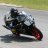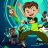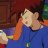# Fibonacci Pivot Points for ThinkOrSwim#### korygill

##### Member
VIP
Warehouse
This study is the thinkorswim version of the same study for Interactive Brokers.

The description of the study is found here:
https://www.interactivebrokers.com/en/software/tws/usersguidebook/technicalanalytics/fibonaccipivotpoints.htm

This is what it looks like on the IB chartThis is what it looks like on the thinkorswim chartThe code is the fibs based on prev day close (could change to weekly, monthly if desired with some modification).

Pivot point studies highlight prices considered to be a likely turning point when looking at values from a previous period, whether it be daily, weekly, quarterly or annual. Each pivot point study has its own characteristics on how these points are calculated. The formula applied for the Fibonacci Pivot Points is:

Pivot Point (P) = (High + Low + Close)/3
Support 1 (S1) = P - {.382 * (High - Low)}
Support 2 (S2) = P - {.618 * (High - Low)}
Support 3 (S3) = P - {1 * (High - Low)}
Resistance 1 (R1) = P + {.382 * (High - Low)}
Resistance 2 (R2) = P + {.618 * (High - Low)}
Resistance 3 (R3) = P + {1 * (High - Low)}

### thinkScript code

Code:
``````# IB_Fib_Pivots
# Author: Kory Gill, @korygill
#
# VERSION HISTORY (sortable date and time (your local time is fine), and your initials
# 20190906-1900-KG    - Created.
# ...
# ...

declare hide_on_daily;
declare once_per_bar;

input AggregationPeriod = AggregationPeriod.DAY;

#
# logic
#
def nan = Double.NaN;
def isRollover = GetYYYYMMDD() != GetYYYYMMDD();
def beforeStart = GetTime() < RegularTradingStart(GetYYYYMMDD());
def afterEnd = GetTime() > RegularTradingEnd(GetYYYYMMDD());
def firstBarOfDay = if (beforeStart == 1 and beforeStart == 0) or (isRollover and beforeStart == 0) then 1 else 0;
def lastBarOfDay = if
(afterEnd[-1] == 1 and afterEnd == 0) or
(isRollover[-1] and firstBarOfDay[-1])
then 1
else 0;

#
# https://www.interactivebrokers.com/en/software/tws/usersguidebook/technicalanalytics/fibonaccipivotpoints.htm
# Pivot Point (P) = (High + Low + Close)/3
# Support 1 (S1) = P - {.382 * (High - Low)}
# Support 2 (S2) = P - {.618 * (High - Low)}
# Support 3 (S3) = P - {1 * (High - Low)}
# Resistance 1 (R1) = P + {.382 * (High - Low)}
# Resistance 2 (R2) = P + {.618 * (High - Low)}
# Resistance 3 (R3) = P + {1 * (High - Low)}
#

def pc = close(period = AggregationPeriod);
def ph = high(period = AggregationPeriod);
def pl = low(period = AggregationPeriod);

#Pivot Point (P) = (High + Low + Close)/3
#Support 1 (S1) = P - {.382 * (High - Low)}
#Support 2 (S2) = P - {.618 * (High - Low)}
#Support 3 (S3) = P - {1 * (High - Low)}
#Resistance 1 (R1) = P + {.382 * (High - Low)}
#Resistance 2 (R2) = P + {.618 * (High - Low)}
#Resistance 3 (R3) = P + {1 * (High - Low)}

def delta = ph - pl;
def pp = if firstBarOfDay then (pc+ph+pl)/3 else if lastBarOfDay then nan else pp;
def s1 = if firstBarOfDay then pp - (.382 * delta) else if lastBarOfDay then nan else s1;
def s2 = if firstBarOfDay then pp - (.618 * delta) else if lastBarOfDay then nan else s2;
def s3 = if firstBarOfDay then pp - (1 * delta) else if lastBarOfDay then nan else s3;
def r1 = if firstBarOfDay then pp + (.382 * delta) else if lastBarOfDay then nan else r1;
def r2 = if firstBarOfDay then pp + (.618 * delta) else if lastBarOfDay then nan else r2;
def r3 = if firstBarOfDay then pp + (1 * delta) else if lastBarOfDay then nan else r3;

plot ppp = pp;
plot ps1 = s1;
plot ps2 = s2;
plot ps3 = s3;
plot pr1 = r1;
plot pr2 = r2;
plot pr3 = r3;

ppp.SetDefaultColor(Color.Red);
ps1.SetDefaultColor(Color.Green);
ps2.SetDefaultColor(Color.White);
ps3.SetDefaultColor(Color.Magenta);
pr1.SetDefaultColor(Color.Yellow);
pr2.SetDefaultColor(Color.Violet);
pr3.SetDefaultColor(Color.Blue);``````

http://tos.mx/xPiy57

•tenacity11, markos and BenTen
R

#### RDMercer

##### New member
This study is the thinkorswim version of the same study for Interactive Brokers.

The description of the study is found here:
https://www.interactivebrokers.com/en/software/tws/usersguidebook/technicalanalytics/fibonaccipivotpoints.htm

This is what it looks like on the IB chartThis is what it looks like on the thinkorswim chartThe code is the fibs based on prev day close (could change to weekly, monthly if desired with some modification).

Pivot point studies highlight prices considered to be a likely turning point when looking at values from a previous period, whether it be daily, weekly, quarterly or annual. Each pivot point study has its own characteristics on how these points are calculated. The formula applied for the Fibonacci Pivot Points is:

Pivot Point (P) = (High + Low + Close)/3
Support 1 (S1) = P - {.382 * (High - Low)}
Support 2 (S2) = P - {.618 * (High - Low)}
Support 3 (S3) = P - {1 * (High - Low)}
Resistance 1 (R1) = P + {.382 * (High - Low)}
Resistance 2 (R2) = P + {.618 * (High - Low)}
Resistance 3 (R3) = P + {1 * (High - Low)}

### thinkScript code

Code:
``````# IB_Fib_Pivots
# Author: Kory Gill, @korygill
#
# VERSION HISTORY (sortable date and time (your local time is fine), and your initials
# 20190906-1900-KG    - Created.
# ...
# ...

declare hide_on_daily;
declare once_per_bar;

input AggregationPeriod = AggregationPeriod.DAY;

#
# logic
#
def nan = Double.NaN;
def isRollover = GetYYYYMMDD() != GetYYYYMMDD();
def beforeStart = GetTime() < RegularTradingStart(GetYYYYMMDD());
def afterEnd = GetTime() > RegularTradingEnd(GetYYYYMMDD());
def firstBarOfDay = if (beforeStart == 1 and beforeStart == 0) or (isRollover and beforeStart == 0) then 1 else 0;
def lastBarOfDay = if
(afterEnd[-1] == 1 and afterEnd == 0) or
(isRollover[-1] and firstBarOfDay[-1])
then 1
else 0;

#
# https://www.interactivebrokers.com/en/software/tws/usersguidebook/technicalanalytics/fibonaccipivotpoints.htm
# Pivot Point (P) = (High + Low + Close)/3
# Support 1 (S1) = P - {.382 * (High - Low)}
# Support 2 (S2) = P - {.618 * (High - Low)}
# Support 3 (S3) = P - {1 * (High - Low)}
# Resistance 1 (R1) = P + {.382 * (High - Low)}
# Resistance 2 (R2) = P + {.618 * (High - Low)}
# Resistance 3 (R3) = P + {1 * (High - Low)}
#

def pc = close(period = AggregationPeriod);
def ph = high(period = AggregationPeriod);
def pl = low(period = AggregationPeriod);

#Pivot Point (P) = (High + Low + Close)/3
#Support 1 (S1) = P - {.382 * (High - Low)}
#Support 2 (S2) = P - {.618 * (High - Low)}
#Support 3 (S3) = P - {1 * (High - Low)}
#Resistance 1 (R1) = P + {.382 * (High - Low)}
#Resistance 2 (R2) = P + {.618 * (High - Low)}
#Resistance 3 (R3) = P + {1 * (High - Low)}

def delta = ph - pl;
def pp = if firstBarOfDay then (pc+ph+pl)/3 else if lastBarOfDay then nan else pp;
def s1 = if firstBarOfDay then pp - (.382 * delta) else if lastBarOfDay then nan else s1;
def s2 = if firstBarOfDay then pp - (.618 * delta) else if lastBarOfDay then nan else s2;
def s3 = if firstBarOfDay then pp - (1 * delta) else if lastBarOfDay then nan else s3;
def r1 = if firstBarOfDay then pp + (.382 * delta) else if lastBarOfDay then nan else r1;
def r2 = if firstBarOfDay then pp + (.618 * delta) else if lastBarOfDay then nan else r2;
def r3 = if firstBarOfDay then pp + (1 * delta) else if lastBarOfDay then nan else r3;

plot ppp = pp;
plot ps1 = s1;
plot ps2 = s2;
plot ps3 = s3;
plot pr1 = r1;
plot pr2 = r2;
plot pr3 = r3;

ppp.SetDefaultColor(Color.Red);
ps1.SetDefaultColor(Color.Green);
ps2.SetDefaultColor(Color.White);
ps3.SetDefaultColor(Color.Magenta);
pr1.SetDefaultColor(Color.Yellow);
pr2.SetDefaultColor(Color.Violet);
pr3.SetDefaultColor(Color.Blue);``````

http://tos.mx/xPiy57
This code does not seem to plot correctly?#### korygill

##### Member
VIP
Warehouse
@RDMercer are you saying the two screenshots I included in the original post do not work for you, or you found some condition where the study does not work? Your post gives no insight into what does not work for you.

•BenTen
R

#### RDMercer

##### New member
@RDMercer are you saying the two screenshots I included in the original post do not work for you, or you found some condition where the study does not work? Your post gives no insight into what does not work for you.
The study seems not to function over all time frames other than intra day or short time frame integration. (1min, 5min, etc) It doesn't work for a 1 y 1d or Wk or larger time frame. Granted, this mat be as designed / intended, might not have every setting 100% correct, or perhaps I misunderstood the study functionality to start with.#### korygill

##### Member
VIP
Warehouse
Ahh...I put this in the original post

The code is the fibs based on prev day close (could change to weekly, monthly if desired with some modification).
It would take more work to have this work on all charts/time frames.

•RDMercerCustom 16Custom 11Questions 5